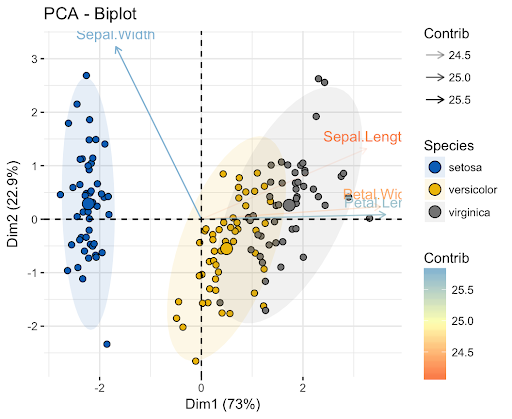# Introduction to Principal Component Analysis

Hey - Nick here! This page is a free excerpt from my new eBook Pragmatic Machine Learning, which teaches you real-world machine learning techniques by guiding you through 9 projects.

In this tutorial, you will receive your first theoretical introduction to principal component analysis.

## What is Principal Component Analysis?

Principal component analysis is an unsupervised machine learning technique that is used to examine the interrelations between sets of variables.

Said differently, principal component analysis studies sets of variables in order to identify the underlying structure of those variables.

Principal component analysis is sometimes called factor analysis.

## The Differences Between Linear Regression and Principal Component Analysis

Based on the description I provided in the last section of this tutorial, you might think that principal component analysis is quite similar to linear regression.

That is not the case. In fact, these two techniques have some important differences.

Linear regression determines a line of best fit through a data set. Principal component analysis determines several orthogonal lines of best fit for the data set.

If you're unfamiliar with the term `orthogonal`, it simply means that the lines are at right angles (90 degrees) to each other.Take a look at the axis labels in this image.

In this image, the x-axis principal component examples 73% of the variance in the data set. The y-axis principal component explains about 23% of the variance in the data set.

This means that 4% of the variance in the dat set remains unexplained. You could reduce this number further by adding more principal components to your analysis.

This will make more sense once we explore a real-world example of principal component analysis in our next tutorial. In fact, I will be revisiting the theory behind principal component analysis several times in the next lesson to solidify your understanding.

## Final Thoughts

In this tutorial, you learned the theoretical underpinnings of principal component analysis. You'll learn about how to build principal component analysis models in Python in our next lesson.

Here's a brief summary of what we covered today:

• That principal component analysis attempts to find orthogonal factors that determine the variability in a data set
• The differences between principal component analysis and linear regression
• What the orthogonal principal components look like when visualized inside of a data set
• That adding more principal components can help you to explain more of the variance in a data set